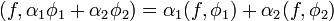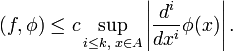# Distribution (mathematics)

Distributions (or generalized functions) are mathematical objects that allow the extension of the concept of the derivative to a much larger class of (not necessarily continuous) functions. Many classical calculus tools, such as convolution or Fourier transform, can be defined for distributions and, more importantly, the theory of differential equations can be developed. This provides the theoretical background for many important non-continuous problems in physics and engineering. In fact, engineers and physicists performed computations using distributions even before the complete mathematical theory was formulated. Using a few properties of otherwise mysterious objects they were able to find the right answers to some practical problems. Now, the spaces of distributions, called Sobolev spaces, are a fundamental concept both in theoretical and applied sciences.

A well known example of a distribution is the Dirac delta. In physics, it may represent a mass distribution (a useful idealization). In signal theory and engineering it is used as an approximate (idealised) model for an "impulse" or "flash" signal: a very short and strong excitation of an electrical circuit. In mathematics, it can be thought of as of the "derivative" of the Heaviside function, a function that is constant everywhere, but makes the unit jump at x = 0. Obviously, if the "derivative" is understood in the classical way as the rate of change, it is infinite at this point (formally, the Heaviside function is not differentiable).

A widely used intuitive description of the Dirac delta reads "this is a function that is equal to zero everywhere except for x = 0; the integral of this function is equal to 1". Strictly speaking, these two intuitive properties are contradictory. It does not matter what value is put at x = 0, the integral of such a function will always be equal to zero. Moreover, if one puts "$\scriptstyle\infty$", as it is often stipulated, then a serious problem arises in applications. For instance, when one deals with signals represented as δ and 2δ say, how can one make a practical difference between$\scriptstyle\infty$ and$2\, \infty$? Definitely, Dirac's delta is not a usual function (strictly speaking, it is not defined on the real line) and a rigorous mathematical background for such an object is therefore essential.

Dirac's intuitive ideas were placed on firm mathematical footing by S. L. Sobolev in 1936, who studied the uniqueness of solutions of the Cauchy problem for linear hyperbolic equations. In 1950 Laurent Schwartz published his Théorie des Distributions. In this book he systematizes the theory of generalized functions unifying all earlier approaches and extending the results.

Nowadays, the physicist's definition of the Dirac delta function$\int_{-\infty}^{\infty} \, \delta(x-x_0) \phi(x)\, dx = \phi(x_0),$

is recognized by the mathematician as a linear functional acting on a set of "well-behaved" test functions φ(x).

In order to understand and generalize this, the concept of "test functions" is needed. Let the set K consist of all real functions φ(x) with continuous derivatives of all orders and bounded support. This means that the function φ(x) vanishes outside some bounded region, which may differ for different functions. The set K is the space of test functions. It can be shown that K is a linear space.

Secondly, the concept of linear functional is needed. We call f a continuous linear functional on K, if f maps all elements of K onto a real number (f, φ) such that

(i). For any two real numbers α1 and α2 and any two functions in K, φ1 and φ2, we have linearity$\, (f,\alpha_1 \phi_1 + \alpha_2 \phi_2) = \alpha_1 (f,\phi_1) + \alpha_2 (f, \phi_2)$

(ii). If the sequence φ1, φ2, … φν, … converges to zero in K (i.e. functions φi have support contained in a common compact set A and the functions and their derivatives converge to 0 in the supremum norm) then$(f,\phi_1), (f, \phi_2), \ldots, (f,\phi_\nu), \ldots$

converges to zero (this is continuity of f). Equivalently, the functional f on K is continuous if for any compact set A ⊂ ℝ there exist a c ∈ ℝ and a k ∈ ℕ such that for any test function φ with support in A$(f,\phi) \le c \sup_{i \le k,\;x\in A} \left| \frac{d^i}{dx^i}\phi(x)\right|.$

A distribution (generalized function) is defined as any linear continuous functional on K.